Download Presentation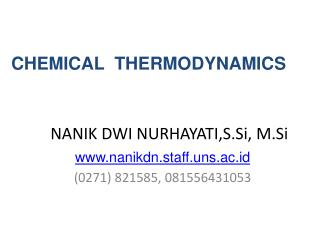NANIK DWI NURHAYATI,S.Si, M.Si

# NANIK DWI NURHAYATI,S.Si, M.Si - PowerPoint PPT Presentation

CHEMICAL THERMODYNAMICS. NANIK DWI NURHAYATI,S.Si, M.Si. www.nanikdn.staff.uns.ac.id (0271) 821585, 081556431053. Enthalpy and Enthalpy Change of Chemical Reactions.I am the owner, or an agent authorized to act on behalf of the owner, of the copyrighted work described.
Download Presentation## NANIK DWI NURHAYATI,S.Si, M.Si

An Image/Link below is provided (as is) to download presentation

Download Policy: Content on the Website is provided to you AS IS for your information and personal use and may not be sold / licensed / shared on other websites without getting consent from its author.While downloading, if for some reason you are not able to download a presentation, the publisher may have deleted the file from their server.

- - - - - - - - - - - - - - - - - - - - - - - - - - E N D - - - - - - - - - - - - - - - - - - - - - - - - - -
Presentation Transcript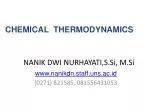CHEMICAL THERMODYNAMICS

### NANIK DWI NURHAYATI,S.Si, M.Si

www.nanikdn.staff.uns.ac.id

(0271) 821585, 081556431053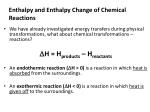Enthalpy and Enthalpy Change of Chemical Reactions
• We have already investigated energy transfers during physical transformations, what about chemical transformations – reactions?

H = Hproducts – Hreactants

• An endothermic reaction (H > 0) is a reaction in which heat is absorbed from the surroundings.
• An exothermic reaction (H < 0) is a reaction in which heat is given off to the surroundings.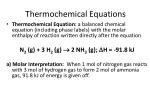Thermochemical Equations
• Thermochemical Equation: a balanced chemical equation (including phase labels) with the molar enthalpy of reaction written directly after the equation

N2 (g) + 3 H2 (g)  2 NH3 (g); H = -91.8 kJ

a) Molar Interpretation: When 1 mol of nitrogen gas reacts with 3 mol of hydrogen gas to form 2 mol of ammonia gas, 91.8 kJ of energy is given off.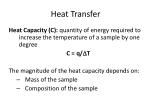Heat Transfer

Heat Capacity (C): quantity of energy required to increase the temperature of a sample by one degree

C = q/T

The magnitude of the heat capacity depends on:

• Mass of the sample
• Composition of the sample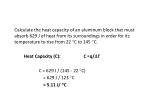Calculate the heat capacity of an aluminum block that must absorb 629 J of heat from its surroundings in order for its temperature to rise from 22 C to 145 C.

Heat Capacity (C): C =q/T

C = 629 J / (145 - 22 C)

= 629 J / 123 C

= 5.11 J/ C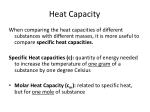Heat Capacity

When comparing the heat capacities of different substances with different masses, it is more useful to compare specific heat capacities.

Specific Heat capacities (c): quantity of energy needed to increase the temperature of one gram of a substance by one degree Celsius

• Molar Heat Capacity (cm): related to specific heat, but for one mole of substance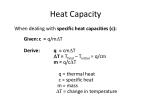Heat Capacity

When dealing with specific heat capacities (c):

Given: c = q/mT

Derive: q = cmT

T = Tfinal – Tinitial = q/cm

m = q/cT

q = thermal heat

c = specific heat

m = mass

T = change in temperature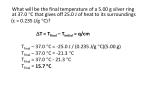What will be the final temperature of a 5.00 g silver ring at 37.0 C that gives off 25.0 J of heat to its surroundings

(c = 0.235 J/g C)?

T = Tfinal – Tinitial = q/cm

Tfinal – 37.0 C = -25.0 J / (0.235 J/g C)(5.00 g)

Tfinal – 37.0 C = -21.3 C

Tfinal = 37.0 C - 21.3 C

Tfinal = 15.7 C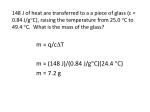148 J of heat are transferred to a a piece of glass (c = 0.84 J/gC), raising the temperature from 25.0 C to 49.4 C. What is the mass of the glass?

m = q/cT

m = (148 J)/(0.84 J/gC)(24.4 C)

m = 7.2 g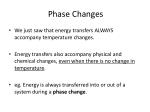Phase Changes
• We just saw that energy transfers ALWAYS accompany temperature changes.
• Energy transfers also accompany physical and chemical changes, even when there is no change in temperature.
• eg. Energy is always transferred into or out of a system during a phase change.Melting/Freezing

Heat of Fusion: quantity of thermal energy that must be transferred to a solid as it melts (qfusion = - qfreezing)

Water:

Heat of fusion =

+333 J/g at 0 C.

Specific Heat (l) =

1.00 cal/gC

Specific Heat (s) depends on T:

 0.5 cal/gC

near 0 C